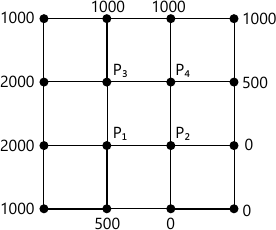MORE IN Engineering Mathematics 3
VTU Computer Science (Semester 3)
Engineering Mathematics 3
June 2015
Total marks: --
Total time: --
INSTRUCTIONS
(1) Assume appropriate data and state your reasons
(2) Marks are given to the right of every question
(3) Draw neat diagrams wherever necessary

1 (a) Expand f(x)=x sin x as a Fourier series in the interval (-π.π), Hence deduce the following
i)$\dfrac{\pi}{2}=1+\dfrac{2}{1.3}-\dfrac{2}{3.5}+\dfrac{2}{5.7}$
ii}$\dfrac{\pi-2}{4}=\dfrac{1}{1.3}-\dfrac{1}{3.5}+\dfrac{1}{5.7}-+....$
7 M
1 (b) Find the half-range Fourier cosine series for the function
$f(x)=\left\{\begin{matrix} kx, &0\leq x\leq l/2 \\k(l-x,) &l/2 where k is non-interger positive constant 6 M 1 (c) Find the constant term and the first two harmonics in the Fourier series for f(x) given by the following table.  X: 0 π/3 2 π3 π 4π/3 5 π/3 2 π F(x): 1 1.4 1.9 1.7 1.5 1.2 0 7 M 2 (a) Find the Fourier transform of the function f(x)=xe-2x 7 M 2 (b) Find the Fourier sine transforms of the Functions \[f(x)\left\{\begin{matrix} Sin x, &0 6 M 2 (c) Find the inverse Fourier sine Transform of \[F(\alpha)=\dfrac{1}{\alpha}e^{-3 \alpha} a>0$
7 M

3 (a) Find the various possible solution of one dimensional wave equation $\dfrac{\partial^2 u}{\partial x^2}=C^2\dfrac{\partial^2 u}{\partial x^2}$ by separable variable method
7 M
3 (b) Obtain solution of heat equation $\dfrac{\partial^2u }{\partial x^2}+\dfrac{\partial^2u }{\partial y^2}=0 \ subject\ to \ condition\ u(0,y)=u(l,y)=0,u(x,0)=0,u(x,s)=\sin \left ( \dfrac{\pi x}{l} \right )$
7 M

4 (a) The pressure P and Volume V of gas are related by the equation PVr = K, where r and k are constant. Fit this equation to the following set of observation (in appropriate units)
 P: 0.5 1 1.5 2 2.5 3 V: 1.62 1 0.75 0.62 0.52 0.46
7 M
4 (b) Solve the following LPP by using the Graphical method:
Maximize: Z=3x1+4x2
Under the Constraints 4x1+2x2≤80
2x1 +5x2≤ 180
x1, x2≥0
6 M
4 (c) Solve the following using simple method
Maximize : Z=2x+4y, subject to the
constraint : 3x+y≤2z, 2x+3y ≤24, x≥0,y≥0
7 M

5 (a) Using Regular- Falsi method, find a real root (correct to three decimal places) of the equation cos x=3x-1 that lies between 0.5 and 1 (Here,x in radians)
7 M
5 (c) Using the power method find the largest energy value corresponding Eigen vectors of the matrix
$\begin{bmatrix} 6 &-2 &2 \\-2 &3 &-1 \\2 &-1 &3 \end{bmatrix}$
taking [1,1,1,]Tas the initial Eigen vectors perform 5 iterations.
7 M

6 (a) From the data given in the following Table; find the number of student who obtained
i) Less than 45 marks ii) Between 40 and 45 marks
 Marks 30-40 40-50 50-60 60-70 70-80 No. of Students 31 42 51 35 31
7 M
6 (b) Using the Lagrange's formula, find the interpolating polynomial that approximates to the function described by the following table
 X 0 1 2 3 4 f(x) 3 6 11 18 27

Hence find f(0.5) and (3.1)
6 M
6 (c) Evaluate $\int_{0}^{1}\limits \dfrac{x}{1+x^2}dx$ by using Simpson's (3/8)th Rule, dividing interval into 3 equal parts Hence find an approximate value of log &sqrt;2.
7 M

7 (a) Solve the one-dimensional wave equation $\dfrac{\partial^2u }{\partial x^2}=\dfrac{\partial^2u }{\partial t^2}$
subject to the boundary conditions u(0,t)=0, u(1,t)=0,t≥0 and the initial conditions $u(x,0)=\sin \pix,\dfrac{\partial t}{\partial t}(x,0)=0 7 M 7 (b) Consider the heat equation \[2\dfrac{\partial^2 u}{\partial x^2}=\dfrac{\partial u}{\partial t}$ under the following conditions:
i) u(0,t)=u(4,t)=0,t≥0
ii) u(x,0)=x(4-x),0 Employ the Bender-Schmidt method with h=1 to find the solution of the equation for 0
6 M
7 (c) Solve the two-dimensional Laplace equation $\dfrac{\partial^2 u}{\partial x^2}=\dfrac{\partial^2 u}{\partial y^2}=0$ at the interior pivotal point of the square region shown in the following figure. The values of u at the pivotal points on the boundary are also shown in the figure7 M

8 (a) State and prove the recurrence relation of Z-Transformation hence find ZT (nP) and $Z_{r}\left [\cos h\left ( \dfrac{n \pi}{2}+0 \right ) \right ]$
7 M
8 (b) Find $Z_{T}^{-1}\left [ \dfrac {z^3-20z}{(z-2)^3 (z-4)} \right ]$
6 M
8 (c) Solve the difference equation
yn+2 -2y n+1 -3yn=3n+2n
Given y0=y1=0
7 M

More question papers from Engineering Mathematics 3